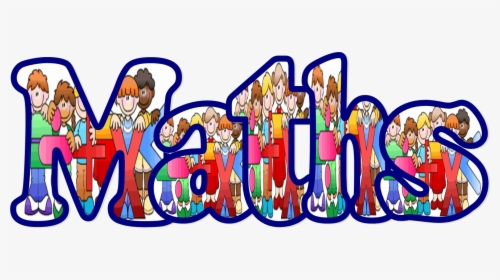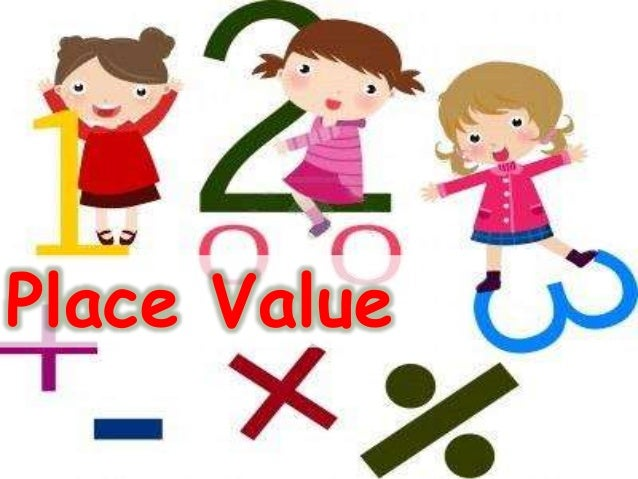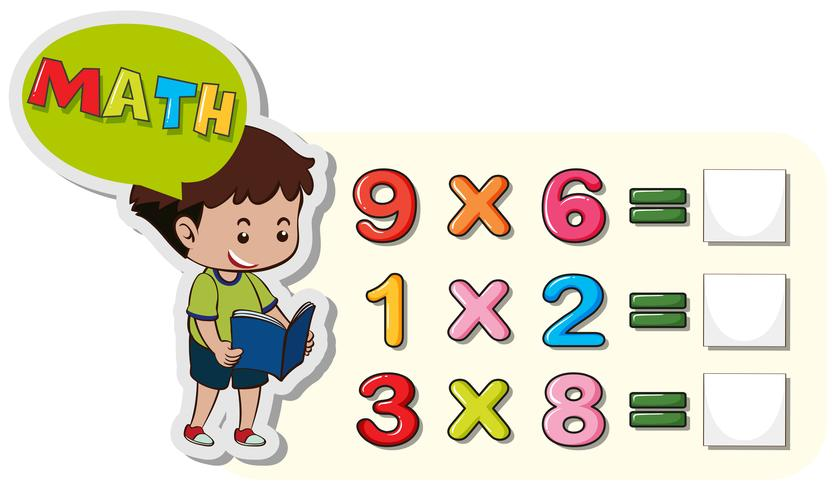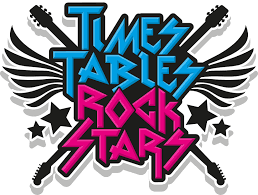# Year 3Please select a calculation area at the side to see step by step videos of the methods we use in our Maths lessons. These videos are useful for both parents and children.

We are adding new videos all the time, so please keep checking.

Useful websitesTargetCount in 1s, 10s, 100s from a given number.

Find 10 or 100 more or less than a given number.

Learn how to find 100 more or less

Practise counting in 10s and 100s activity

Counting in 100s activity

TargetRecognise the place value of each digit in a three digit number (hundreds, tens, ones).

Partition a number knowing the value of each digit (hundred, tens, ones).

Learn how to read and write three digit numbers

Recap how to partition two digit numbers

Learn how to partition three digit numbers

Learn how to order and compare three digit numbers

Recognise the value of each digit activity

Partitioning activity 1

Partitioning activity 2

Partitioning activity 3

Expanded form activity

TargetBe fluent in number bonds to 50 and 100.

Learn about number bonds to 100

Number bonds to 100 activity 1

Number bonds to 100 activity 2

Number bonds within 100 activityTarget - Add and subtract numbers mentally including:

Three digit number and ones

Three digit number and tens

Three digit number and hundreds

Learn how to add tens from a three digit number

Learn how to subtract tens from a three digit number

Subtracting tens and hundreds activityTarget - Recall and use multiplication and division facts for 3, 4, 6 and 8 times tables

Learn how to multiply and divide by 3

3 times table song

Learn how to multiply and divide by 4

4 times table song

Learn how to multiply and divide by 6

6 times table song

Learn how to multiply and divide by 8

8 times table songTT Rock Stars is great way to practise your times tables and enter competitions.

TT Rock Stars

Target - Use known multiplication and division facts to mentally calculate two digit by one digit numbers

Learn how to use arrays to calculate two digit by one digit numbers

Learn how to use dienes to calculate two digit by one digit numbers

Multiply two digit by one digit numbers activity

Target - Doubles and halves to 50

Doubling and halving activity

Daily maths challengeIf you would like to have a go at a daily maths challenge click on the link below.

Daily 10 challenge

Select level three and choose your challenge from any of the options.

Have a go each day and see if you can beat your score from the previous day.  Good luck!